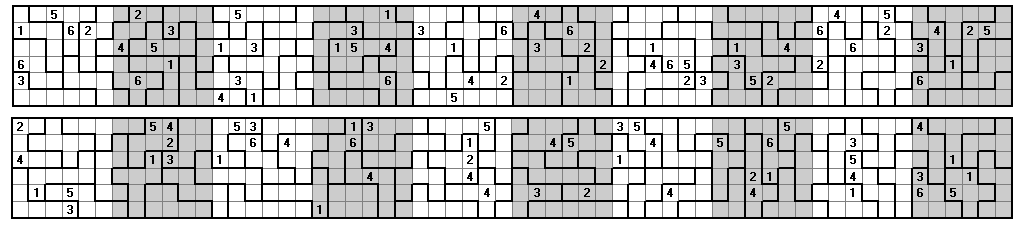Hexomino Sudoku ProblemsTwo Square

The basis of these problems is patterns created from the twelve piece set we get by adding two monominoes to a 2x2 square.Three Square

There are 19 convex tridominoes - omitting the 6x1 rectangle we get a set of 18 pieces which can be used to form three side 6 squares.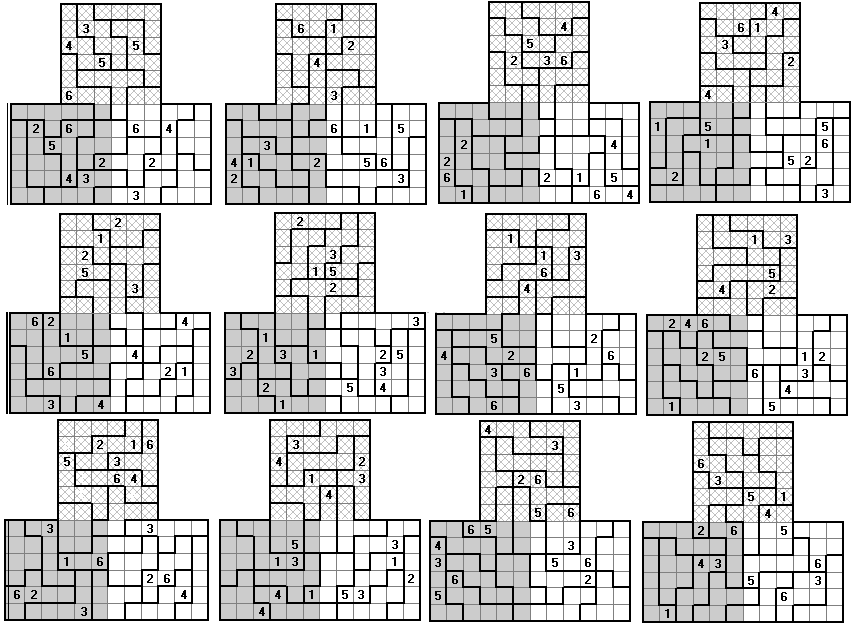Four Square

These problems are based on the 24 balanced hexominoes.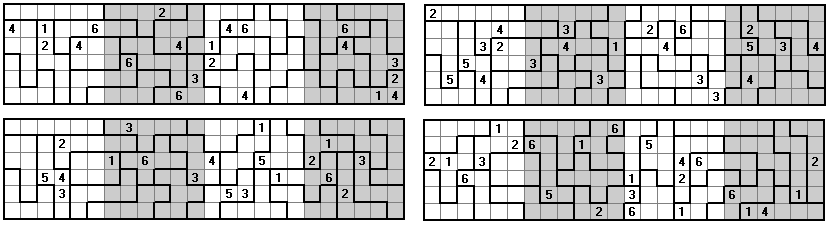These next four problems have the added condition that no equal numbers can adjoin across panel boundaries.Here the pieces form a 12x12 square.Five Square

Here we use the set of thirty pieces using the non-symmetric convex one-sided tridominoes.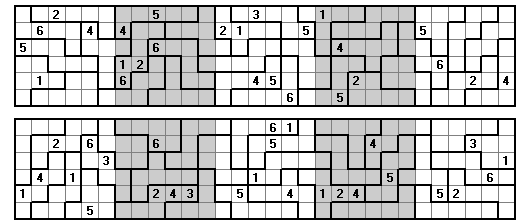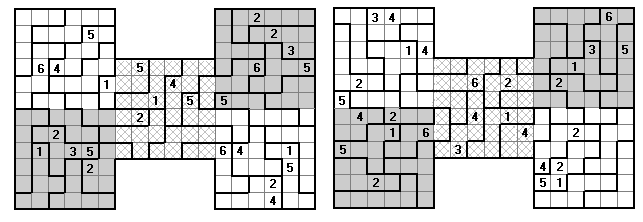Alternatively, we can use the set of hexominoes which can fit into a 4x4 square. This consists of 29 pieces and so one of the omitted pieces is included.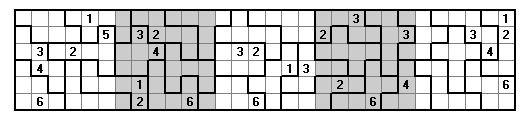The same set with the five squares arranged differently.Another version of the above pattern with only 22 clues.Finally a version with only 19 clues. Although this has a unique solution and is possible by hand it is extremely difficult.One extra clue makes a big difference - that version in hereSix Square

These problems are based on rectangles formed with the 35 hexominoes with one of the unbalanced pieces used twice.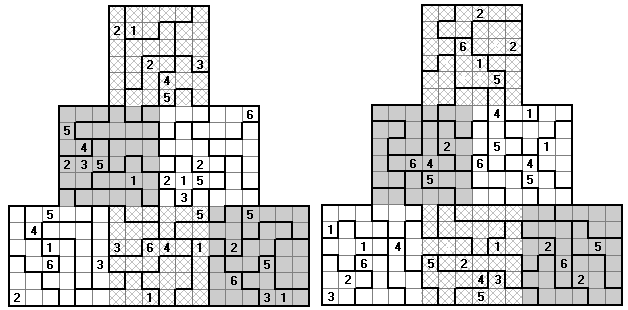Ten Square

These problems are based on a 6x60 rectangle formed with the 60 one-sided hexominoes